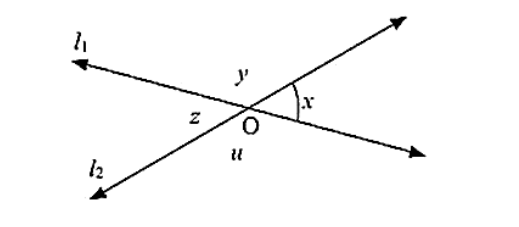# In the below fig, lines l1, and l2 intersect at O, forming angles as shown in the figure. If x = 45. Find the values of x,

Question:

In the below fig, lines l1, and l2 intersect at O, forming angles as shown in the figure. If x = 45. Find the values of x, y. z and u.Solution:

Given that

X = 45°, Y = ?, Z = ?, u = ?

Vertically opposite angles are equal

Therefore z = x = 45

z and u are angles that are a linear pair

Therefore, z + u = 180

z = 180 - u

u = 180 - x

u = 180 - 45

u = 135

x and y angles are a linear pair

x+ y = 180

y = 180 - x

y =180 - 45

y = 135

Hence,  x = 45, y = 135, z = 135  and u = 45